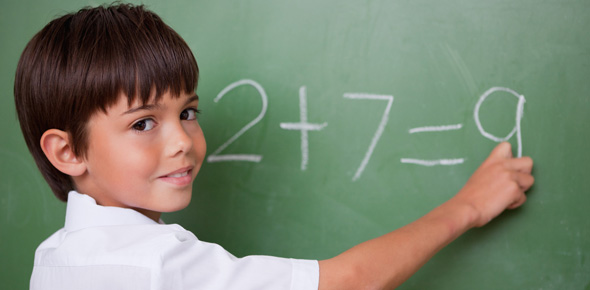# Ei Math Addition And Its Properties

20 QuestionsSettingsMath

Related Topics
• 1.
• A.

When zero is added to a number, the sum is the number itself

• B.

Changing the order of the addends does not change the sum

• C.

Changing the grouping of the addends does not change the sum

• 2.
Which number shows the grouping property of addition?
• A.

2 + 6 = 6 + 2

• B.

8 + 0 = 8

• C.

4 + (2 + 7) = (4 + 2) + 7

• 3.
What is missing in the number sentence 12 + ___ = 12?
• A.

12

• B.

1

• C.

0

• 4.
What property is being shown in the number sentence 3 + 7 = 7 + 3?
• A.

Grouping property

• B.

Order property

• C.

Zero property

• 5.
STORY PROBLEM: The baker made 6 chocolate cakes and baked 4 mango cakes.  How many cakes did he make in all? QUESTION: What is asked in the STORY PROBLEM?
• A.

Number of mango cakes

• B.

Number of chocolate cakes

• C.

Number of cakes

• 6.
STORY PROBLEM: The baker made 6 chocolate cakes and baked 4 mango cakes. How many cakes did he make in all? QUESTION: What is/are the clue word/clue words  in the STORY PROBLEM?
• A.

In all

• B.

How

• C.

How many

• 7.
STORY PROBLEM: The baker made 6 chocolate cakes and baked 4 mango cakes. How many cakes did he make in all? QUESTION: What is the number sentence fit for the problem?
• A.

6 + 3 = N

• B.

6 + 4 = N

• C.

6 + 5 = N

• 8.
What are the missing numbers to make the number sentence TRUE? ___ + 2 = ___ + 5
• A.

2 and 4

• B.

2 and 5

• C.

5 and 2

• D.

4 and 2

• 9.
A baker can make 6 cakes in a day.  How many cake can he bake in 5 days?
• A.

56

• B.

65

• C.

30

• 10.
STORY PROBLEM: The baker made 6 chocolate cakes and baked 4 mango cakes. How many cakes did he make in all? QUESTION? Which number sentence fits the story?
• A.

6 + 4 = N

• B.

6 - 4 = N

• C.

6 x 4 = N

• 11.
STORY PROBLEM: The baker made 6 chocolate cakes and baked 4 mango cakes. How many cakes did he make in all? QUESTION? Which operation would help solve the problem?
• A.

• B.

Subtraction

• C.

Multiplication

• 12.
The numbers that we put together is called ...
• 13.
• A.

True

• B.

False

• 14.
The result of addition is called sum.
• A.

True

• B.

False

• 15.
Check two numbers that will give a sum of 14.
• A.

3

• B.

8

• C.

6

• D.

9

• 16.
Check two numbers that will give a sum of 9.
• A.

2

• B.

3

• C.

5

• D.

6

• 17.
When you add 3 to me, the result is 8.  How do you call the number that you add to another number?
• A.

Plus

• B.

• C.

Sum

• 18.
Addition is a process when you put things together to make a bigger one.
• A.

True

• B.

False

• 19.
Decrease is a clue word in addition.
• A.

True

• B.

False

• 20.
When you add zero to a number the result is the number itself.  This property is called the commutative property of addition.
• A.

True

• B.

False